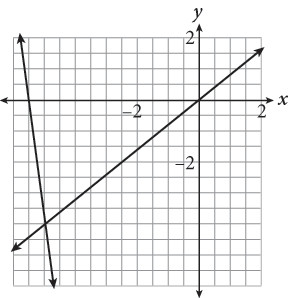# SAT Math Multiple Choice Question 322: Answer and Explanation

### Test Information

Question: 322

7.If (A, B) is the solution to the system of equations shown in the graph above, what is the value of A + B?

• A. –18
• B. –9
• C. 1
• D. 5.5

Strategic Advice: The solution to a system of linear equations shown graphically is the point where the lines intersect. Read the axis labels carefully. Each grid-line represents